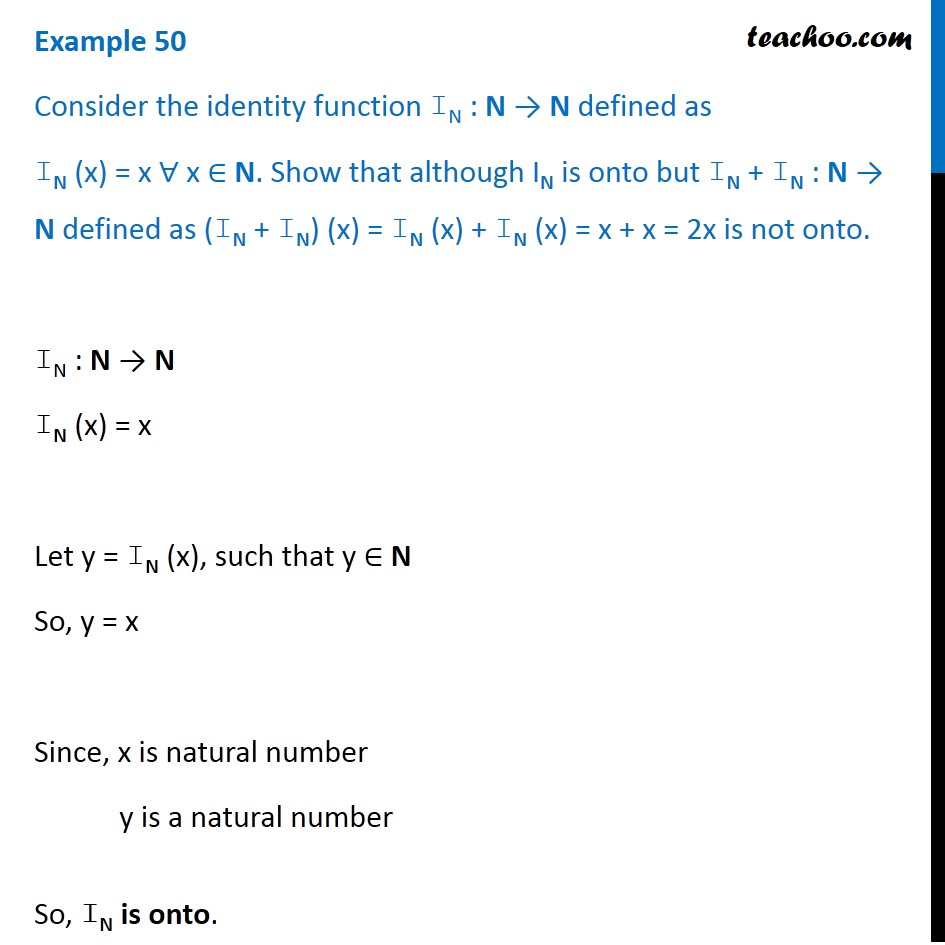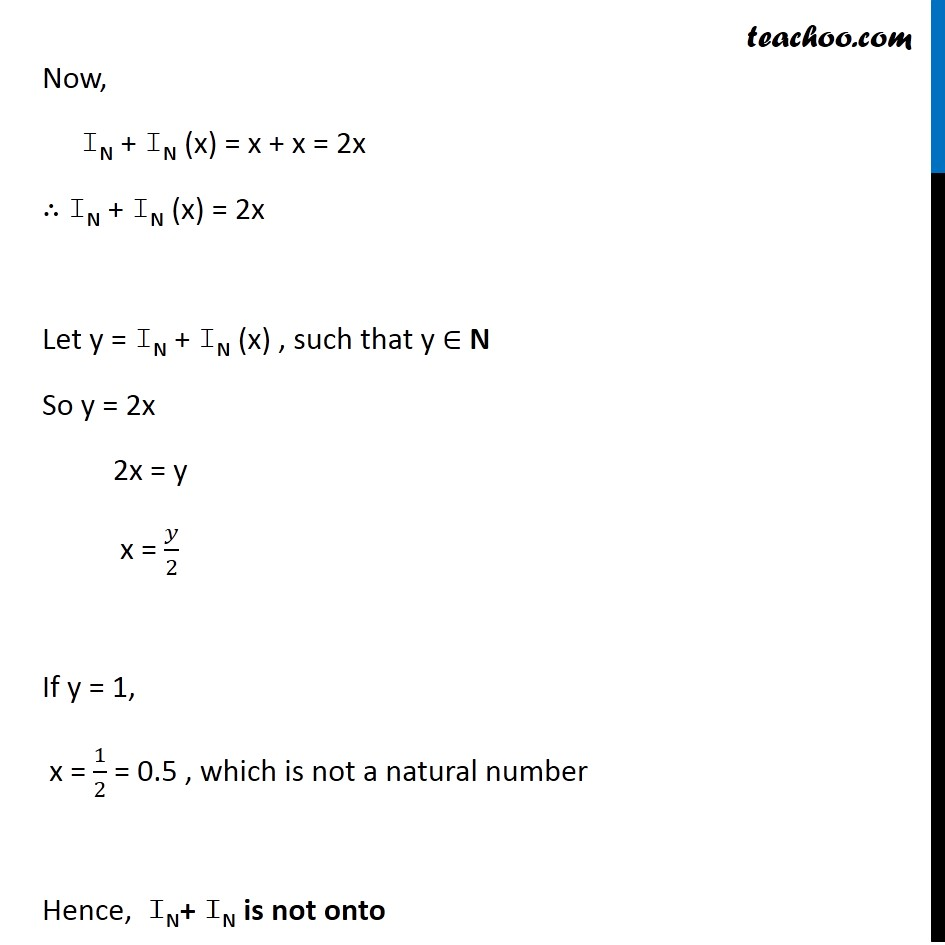1. Chapter 1 Class 12 Relation and Functions (Term 1)
2. Serial order wise
3. Examples

Transcript

Example 50 Consider the identity function IN : N → N defined as IN (x) = x ∀ x ∈ N. Show that although IN is onto but IN + IN : N → N defined as (IN + IN) (x) = IN (x) + IN (x) = x + x = 2x is not onto. IN : N → N IN (x) = x Let y = IN (x), such that y ∈ N So, y = x Since, x is natural number y is a natural number So, IN is onto. Now, IN + IN (x) = x + x = 2x ∴ IN + IN (x) = 2x Let y = IN + IN (x) , such that y ∈ N So y = 2x 2x = y x = 𝑦/2 If y = 1, x = 1/2 = 0.5 , which is not a natural number Hence, IN+ IN is not onto

Examples